# Literals in C#

## Literals in C# with Examples

In this article, I am going to discuss Literals in C# with Examples. Please read our previous article, where we discussed Data Types in C# with Examples. At the end of this article, you will understand what are literals and when and how to use Literals in C# Applications.

##### Literals in C#

The Literals in C# are the fixed values (or hard-coded values) given to your variable and these values cannot be modified during the execution of the program.

1. The fixed values are called Literals in C#.
2. Literal is a value that is used by the variables.

For example, int x = 100; Here x is a variable, and 100 is literal.

##### Types of Literals in C#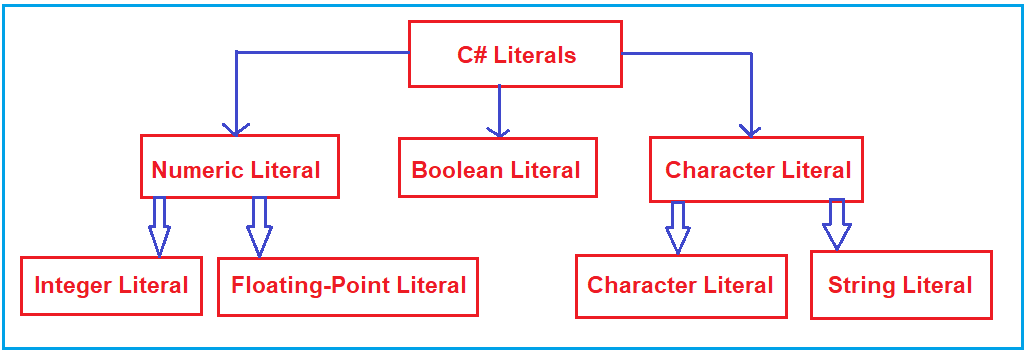As you can see in the above image, the literals are broadly classified into the following five categories.

1. Integer Literals
2. Floating-point Literals
3. Character Literals
4. String Literals
5. Boolean Literals

Let us discuss each of these Literals in C# with Examples.

##### Integer Literals in C#:

The Integer literals in C# are used to write values of types int, uint, long, ulong, etc. We can represent the Integer Literals in the form of Decimal, Binary, or Hexadecimal. Here, we need to use a prefix to specify whether the Integer Literal is Binary (prefixed with 0b), or Hexadecimal (0X) type. No prefix is required for the Decimal numbers.

By default, every Integer Literal is of int type. For Integral data types (byte, short, int, long), we can specify literals or fixed values in the following 3 ways:

1. Decimal (Base-10): Digits from 0-9 are allowed in this form. No prefix is required for the decimal type of literal. Example: int x=101;
2. Hexa-Decimal (Base-16): Digits 0-9 are allowed and also characters from a-f are allowed in this form. Furthermore, both uppercase and lowercase characters can be used. C# provides an exception here i.e. as we know that C# is a case-sensitive programming language but here C# is not case-sensitive. Here, the hexadecimal number should be prefixed with 0X or 0x. and suffix with Face. Example: int x = 0X123F;
3. Binary (0 and 1): In this form, the allowed digits are only 1’s and 0’s. The binary number should be prefixed with 0b. Example: int x = 0b1111;

Note: There is no Octal Number Literals in C#. On many websites, you will find that in Octal number, digits from 0 – 7 are allowed and the octal number should always have a prefix 0. Example: int x=0146; But this is wrong. In C#, Octal Number Representation is not possible. Refer to the following Stack Overflow link.

https://stackoverflow.com/questions/4247037/octal-equivalent-in-c-sharp

##### Example to Understand Integer Literals in C# Language
```using System;
namespace LiteralsDemo
{
class Program
{
static void Main(string[] args)
{
// Decimal literal
//Allowed Digits: 0 to 9
int a = 101; //No suffix is required

// Hexa-Decimal Literal
//Allowed Digits: 0 to 9 and Character a to f
int c = 0x123f; //Prefix with 0x, and suffix with f

//Binary literal
//Allowed Digits: 0 to 1
int d = 0b1111; // //Prefix with 0b

Console.WriteLine(\$"Decimal Literal: {a}");
Console.WriteLine(\$"Hexa-Decimal Literal: {c}");
Console.WriteLine(\$"Binary Literal: {d}");

}
}
}
```
###### Output: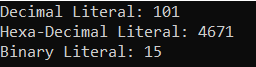To understand how a hexadecimal number is converted to decimal, please check the following website.

A suffix can also be used with the integer literals like U or u are used for unsigned numbers while l or L are used for long numbers. For a better understanding, please have a look at the following example.

```using System;
namespace LiteralsDemo
{
class Program
{
static void Main(string[] args)
{
int a = 1000; //Integer
uint b = 1000U; //Unsigned Integer
long c = 1000L; //Long
ulong d = 1000UL; //Unsigned Long

Console.WriteLine(\$"Integer Literal: {a}");
Console.WriteLine(\$"Unsigned Integer Literal: {b}");
Console.WriteLine(\$"Long Literal: {c}");
Console.WriteLine(\$"Unsigned Long Literal: {d}");

}
}
}
```
###### Output:##### Floating-Point Literals in C#:

The Literals in C# which have an integer part and a decimal point are known as Floating-Point literals i.e. Numbers with Decimal. The Floating-Point Literals are used to write values of types float, double, and decimal.

By default, every floating-point literal is of double type and hence we can’t assign values directly to float and decimal variables. If you want to assign values to a float variable, then you need to add the suffix f at the end of the floating-point literal. Similarly, if you want to assign values to a decimal variable, then you need to add the suffix m or M at the end of the floating-point literal. If you are not suffixing the floating-point literal with anything, then the floating-point literal is going to be double by default. Even, if you want, then you can also specify explicitly floating-point literal as the double type by suffixed with d or D, of course, this convention is not required.

##### Example to Understand Floating-Point Literals in C#:
```using System;
namespace LiteralsDemo
{
class Program
{
static void Main(string[] args)
{
//Double Literal
double a = 10.15; //By Default Floating Point Literal is double

//Float Literal
float b = 100.72F; //Suffix with F

//Double Literal
double c = 1.45D; //Suffix with D

//Decimal Literal
decimal d = 1.44M; //Suffix with M

Console.WriteLine(\$"Double Literal: {a}");
Console.WriteLine(\$"Float Literal: {b}");
Console.WriteLine(\$"Double Literal: {c}");
Console.WriteLine(\$"Decimal Literal: {d}");

}
}
}
```
###### Output: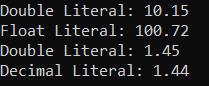##### Character Literals in C#:

The Character Literals in C# are enclosed in single quotes, for example, ‘a’, and can be stored in a simple variable of char data type. A character literal can be a plain character for example, ‘a’, an escape sequence for example, ‘\t’, or a universal character for example, ‘\u02B0’. So, for character data types we can specify character literals in 3 ways. They are as follows:

###### 1. Character Literals using Single Quote:

We can specify Character literals to a char data type as a single character using a single quote.
Example: char ch = ‘A’;

###### 2. Character Literals using Unicode Representation:

We can specify Character literals using Unicode representation ‘\uXXXX’ where XXXX is the 4 hexadecimal numbers.
Example: char ch = ‘\u0041’; // Here /u0041 represent A. Please check the below link for the list of Unicode characters.
https://en.wikipedia.org/wiki/List_of_Unicode_characters

###### 3. Character Literals using Escape Sequence:

Every escape character in C# can be specified as a character literal.
Example: char ch = ‘\n’;
There are certain characters in C# when preceded by a backslash, which will have a special meaning that they are used to represent. For example, newline (\n) and tab (\t). The following is the list of some of the escape sequence characters available in C#.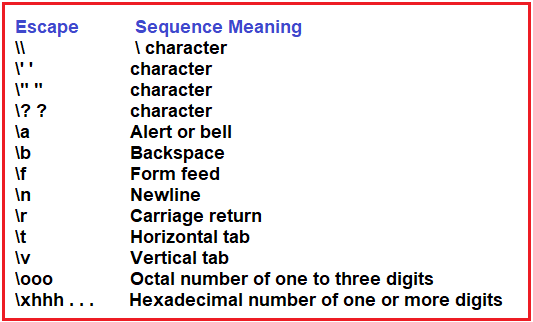##### Example to Understand Character Literals in C#:
```using System;
namespace LiteralsDemo
{
class Program
{
static void Main(string[] args)
{
//Character literal using single quote
char ch1 = 'A';
Console.WriteLine("Single Quote: " + ch1);

//Character literal using Unicode representation
char ch2 = '\u0041';
Console.WriteLine("Unicode: " + ch2);

//Character literal using Escape character
Console.WriteLine("Escape: Hello\nDotNet\tTutorials");

}
}
}
```
###### Output: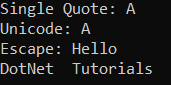##### String Literals in C#:

The Literals in C# which are enclosed with double quotes (” “) or start with @” “ are known as the String literals. In C#, we can represent the string literals in two ways. They are as follows:

1. Regular String Literals: A regular string literal in C# consists of zero or more characters enclosed in double quotes, for example, “Dot Net Tutorials”, and may include both simple escape sequences for example, “Dot\nNet\tTutorials” and Unicode escape sequences.
2. Verbatim String Literals: A verbatim string literal starts with an @ character followed by a double-quote which may contain zero or more characters, and ends with a double-quote character. They can store characters or escape sequences, for example, @”Dot\nNet\tTutorials”. In this case, the escape sequences or characters will be printed as it is in the output.
##### Example to understand String Literals in C#:
```using System;
namespace LiteralsDemo
{
class Program
{
static void Main(string[] args)
{
string str1 = "Dot Net Tutorials";
string str2 = @"Dot Net Tutorials";

string str3 = "Dot\nNet\tTutorials";
string str4 = @"Dot\nNet\tTutorials";

Console.WriteLine(\$"str1: {str1}");
Console.WriteLine(\$"str2: {str2}");
Console.WriteLine(\$"str3: {str3}");
Console.WriteLine(\$"str4: {str4}");

}
}
}
```
###### Output: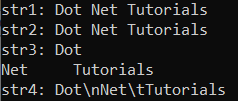##### Boolean Literals in C#:

Only two values are allowed for Boolean literals i.e. true and false. Boolean literals are simple. There are only two logical values that a boolean value can have, true and false. The values of true and false do not convert into any numerical representation. The true literal in C# does not equal 1, nor does the false literal equal 0.

Example:
bool b1 = true;
bool b2 = false;
bool b3 = 0; //Error
bool b4 = 1; //Error

Note: The assigned true and false values should be the lower case only otherwise you will get compile time error. The following is not allowed.
bool b1 = True; //Error
bool b2 = False; //Error
bool b1 = TRUE; //Error
bool b2 = FALSE; //Error

##### Example to understand Boolean Literals in C#:
```using System;
namespace LiteralsDemo
{
class Program
{
static void Main(string[] args)
{
bool b1 = true;
bool b2 = false;
// bool b3 = 0; //Error
// bool b4 = 1; //Error

Console.WriteLine(b1);
Console.WriteLine(b2);
}
}
}
```
###### Output: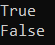##### Binary Literals in C#:

The binary literal is used to store the binary value in a variable. And if you want to create a binary literal, then the literal value should be prefixed with 0b. And here, you can use only 0 and 1. If you use any other number then you will get compile time error.
int num1 = 0b10001; //Allowed
int num2 = 0b1000145; //Error
Here when the compiler sees 0b in the variable value, then it automatically treated this literal as a binary literal.

##### Example to understand Binary Literals:
```using System;
namespace LiteralsDemo
{
class Program
{
static void Main(string[] args)
{
// Creating binary literals by prefixing with 0b
int Num1 = 0b100111101;
int Num2 = 0b01000011;
//int num3 = 0b100134; //Error

Console.WriteLine(\$"Value of Num1 is: {Num1}");
Console.WriteLine(\$"Value of Num2 is: {Num2}");
Console.WriteLine(\$"Char value of Num1 is: {Convert.ToChar(Num1)}");
Console.WriteLine(\$"Char value of Num2 is: {Convert.ToChar(Num2)}");

}
}
}
```
###### Output: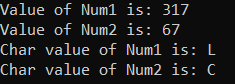Note: C# Binary Literals feature allows us to deal with binary values in the C# application. By using this feature, we can store binary values in variables. C# provides 0b literals to create binary values. C# compiler recognizes these literals and treats values accordingly.

In the next article, I am going to discuss Type Casting in C# with Examples. Here, in this article, I try to explain Literals in C# with Examples. I hope you enjoy this article.

### 2 thoughts on “Literals in C#”

1.why we are referring literals with constant , as its value we can change , for constant we use const keyword..

1.Why we are using readkey at the end